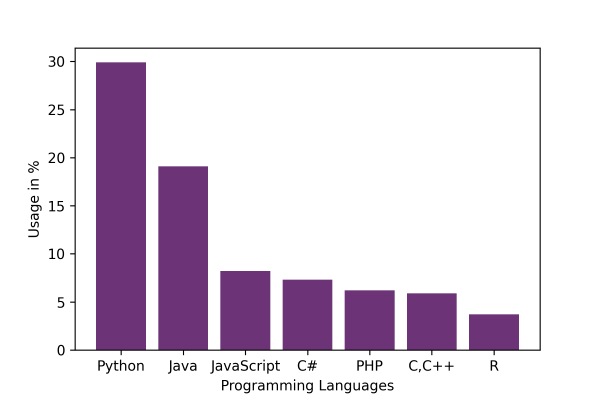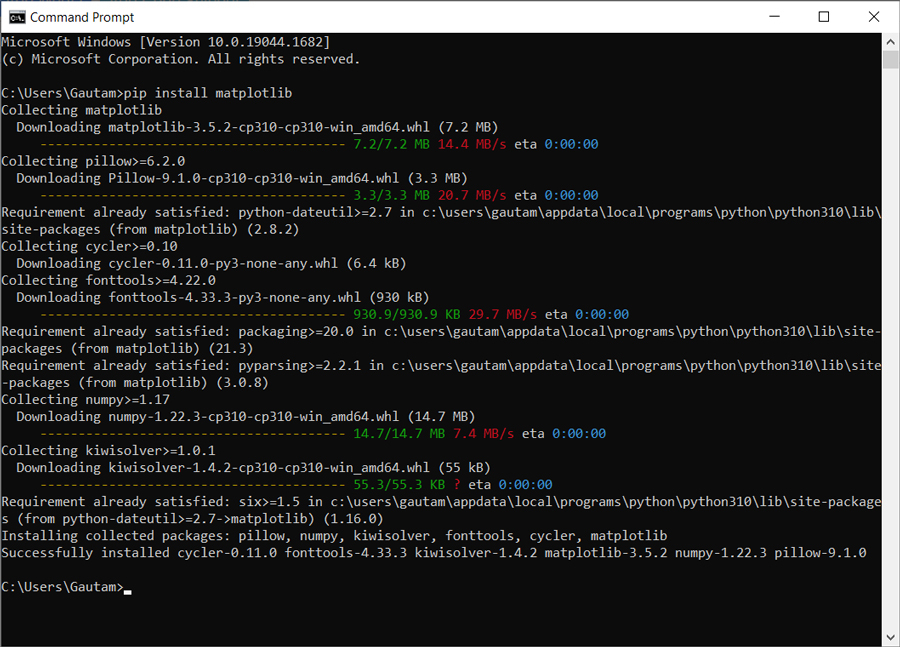## Python Programming Examples Tutorial Index

This Python program contains a simple program to generate bar graphs using the Matplotlib Python library. Matplotlib Python has an extensive library for creating stable, animated, and interactive data visualizations.

In this Python program, we have used the popularity data of different programming languages and displayed it as a bar graph using the Matplotlib Python library.

Example:

An example of a Python Matplotlib Bar Graph:

``````import matplotlib.pyplot as pyplot

# Manual data setup
labels = ('Python', 'Java', 'JavaScript', 'C#', 'PHP', 'C,C++', 'R')
index = (1, 2, 3, 4, 5, 6, 7) # provides locations on x axis
sizes = [29.9, 19.1, 8.2, 7.3, 6.2, 5.9, 3.7]

# bar chart setup
pyplot.bar(index, sizes, color="#6c3376", tick_label=labels)

# layout configuration
pyplot.ylabel('Usage in %')
pyplot.xlabel('Programming Languages')

# Save the chart file
#pyplot.savefig('filename.png', dpi=300)

# Print the chart
pyplot.show()``````

Program Output:### Install Matplotlib

The Matplotlib Python library is installed using pip (package manager for Python). The following command installs the Matplotlib package:

`pip install matplotlib`